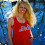## Saturday, October 31, 2015

### 33 76 106 | Michael Conforto's Two Home Runs In Game 4 of 111th World Series, Rookie Sensation for Mets

Michael = 4+9+3+8+1+5+3 = 33
Thomas = 2+8+6+4+1+1 = 22/31
Conforto = 3+6+5+6+6+9+2+6 = 43
Michael Conforto = 76 (MC = 33, Jewish Gematria)
Michael Thomas Conforto = 98/107

Michael Conforto has hit two home runs on October 31, 2015, in Game 4 of the World Series.  The game is not over yet either.

10/31/2015 = 10+31+20+15 = 76

In Simple English Gematria, the name 'Comforto' creates numbers that have been very important to this baseball season.

Michael = 13+9+3+8+1+5+12 = 51
Conforto = 3+15+14+6+15+18+20+15 = 106

Michael was born March 1, 1993, emphasis on '93.

3/1/1993 = 3+1+1+9+9+3 = 26
3/1/1993 = 3+1+19+93 = 116
3/1/93 = 3+1+93 = 97

He is currently 22-years old.  22 has a numerology of 4 (2+2 = 4), and he is making a big impact of Game 4.

His MLB debut has something to do with World Series.

7/24/2015 = 7+24+20+15 = 66
7/24/2015 = 7+24+(2+0+1+5) = 39 (Born in '93)
7/24/2015 = 7+2+4+2+0+1+5 = 21
7/24/15 = 7+24+15 = 46

Conforto was called up 99-days before having his big game in the 111th World Series.  As a child, he played in the Little League World Series.  He is from Woodinville, which is a perfect match for Kansas City Royals.

Woodinville = 5+6+6+4+9+5+4+9+3+3+5 = 59/77 (CitField = 77)
Kansas City Royals = 2+1+5+1+1+1+3+9+2+7+9+6+7+1+3+1 = 59/86

You have to love the '86' Gematria in Kansas City Royals, seeing as how they're going against the Mets, who last won the World Series in '86.

http://www.seattletimes.com/sports/mariners/woodinville-draftee-michael-conforto-signs-with-mets/

#### 1 comment:

1.I had a feeling the Royals would win today because of the date, 10/31. It also matches up with American Pharoah's final win. Tomorrow is not only 11/1, but we will be in the 11th month...so the Mets will prolly pull off the wins the next three games. I don't see how Kansas City winning would be any kind of tribute to New York... :) We'll see...